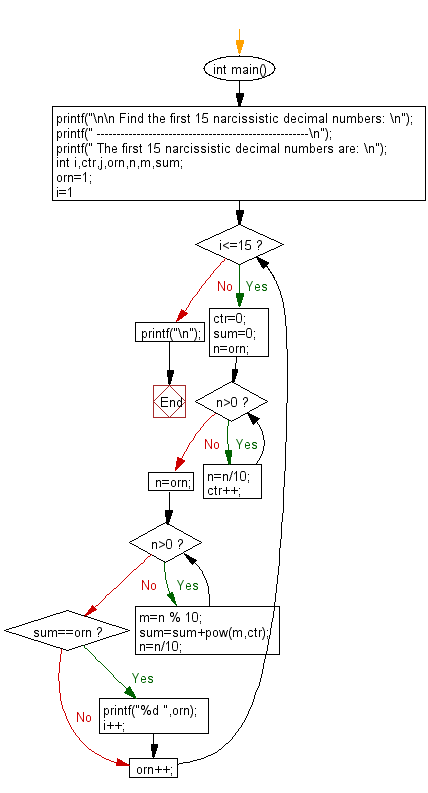﻿ C Program: Show first 15 Narcissistic decimal numbers# C Exercises: Generate and show the first 15 narcissistic decimal numbers

## C Numbers: Exercise-10 with Solution

Write a program in C to generate and show the first 15 narcissistic decimal numbers.

Sample Solution:

C Code:

``````# include <stdio.h>
# include <math.h>
# include <stdlib.h>

int main()
{
printf("\n\n Find the first 15 narcissistic decimal numbers: \n");
printf(" -----------------------------------------------------\n");
printf(" The first 15 narcissistic decimal numbers are: \n");
int i,ctr,j,orn,n,m,sum;
orn=1;
for (i=1;i<=15;)
{
ctr=0;
sum=0;
n=orn;
while(n>0)
{
n=n/10;
ctr++;
}
n=orn;
while(n>0)
{
m=n % 10;
sum=sum+pow(m,ctr);
n=n/10;
}
if(sum==orn)
{
printf("%d ",orn);
i++;
}
orn++;
}
printf("\n");
}
```
```

Sample Output:

``` The first 15 narcissistic decimal numbers are:
1 2 3 4 5 6 7 8 9 153 370 371 407 1634 8208
```

Pictorial Presentation:Flowchart:C Programming Code Editor:

What is the difficulty level of this exercise?

Test your Programming skills with w3resource's quiz.

﻿

## C Programming: Tips of the Day

Why do C and C++ compilers allow array lengths in function signatures when they're never enforced?

It is a quirk of the syntax for passing arrays to functions.

Actually it is not possible to pass an array in C. If you write syntax that looks like it should pass the array, what actually happens is that a pointer to the first element of the array is passed instead.

Since the pointer does not include any length information, the contents of your [] in the function formal parameter list are actually ignored.

Ref : https://bit.ly/3fhlvdH